GAMES101 2020-Spring 对应的 8 次 PA 整理。

Warning: FYI, these codes may be ugly and buggy.

## PA1

### 算法回顾

• 视图变换：定义 $M_{view}$ 变换相机，使得经过该变换满足 $\vec e = \vec 0, \hat g = -\hat z, \hat t = \hat y$，其中：$\vec e$ 为相机所在位置， $\hat g$ 为相机看的方向，$\hat t$ 为相机的向上方向。

• 投影变换

• 近平面的坐标不改变
• 远平面的 $x,y$ 坐标被压缩至与近平面相同
• 近平面中心点与远平面中心点的位置不变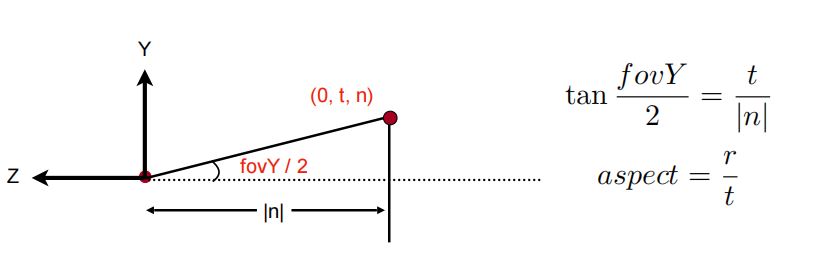### 实验结果## PA2

• rasterize_triangle(): 执行三角形栅格化算法
• static bool insideTriangle(): 测试点是否在三角形内
• 正确实现深度缓冲算法
• Super-sampling

### 算法回顾

• 检测点在三角形内

• Super-sampling

• 深度缓冲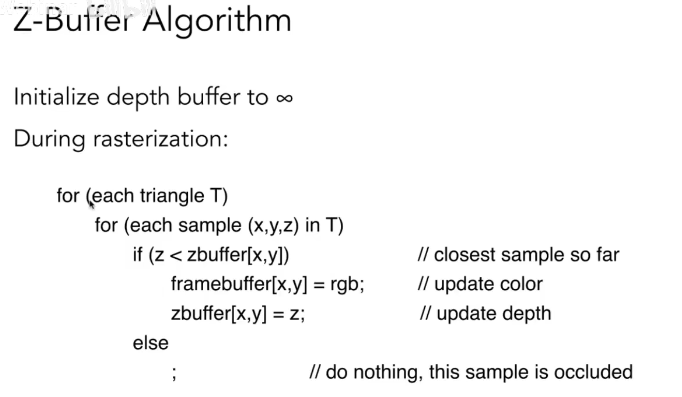### 实验结果## PA3

• 参数插值
• Blinn-Phong 模型
• Texture Mapping
• Bump Mapping & Displacement Mapping
• 尝试更多模型
• 双线性插值

### 算法回顾

• 重心坐标插值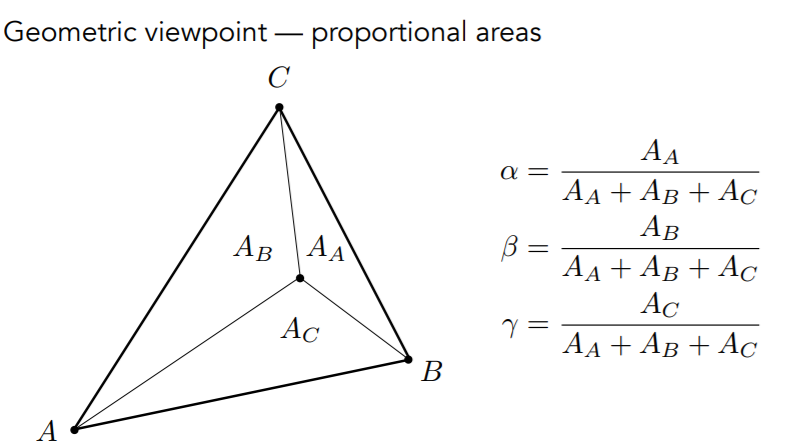• BP

### 实验结果

• Normal• BP• Texture• Bump Mapping & Displacement Mapping• Bunny• 双线性插值前/后 (Shrink texture.png by 50%)### 实验框架

• main.cpp
• Eigen::Matrix4f get_view_matrix(Eigen::Vector3f eye_pos)
• Eigen::Matrix4f get_model_matrix(float angle)
• Eigen::Matrix4f get_projection_matrix(float eye_fov, float aspect_ratio, float zNear, float zFar)
• struct Light {Eigen::Vector3f position; Eigen::Vector3f intensity;};
• Eigen::Vector3f texture_fragment_shader(const fragment_shader_payload& payload)
• Eigen::Vector3f phong_fragment_shader(const fragment_shader_payload& payload)
• 定义 $k_d, k_s, k_a, lights, amb_light, eye_pos$​
• 返回 $result_color * 255.0$
• Eigen::Vector3f displacement_fragment_shader(const fragment_shader_payload& payload)
• Eigen::Vector3f bump_fragment_shader(const fragment_shader_payload& payload)
• int main()
• 读取模型，然后将模型中的各个面建立 Triangle 对象
• 建立 Rasterizer 类对象，设置材质
• 调用渲染函数，然后使用 OpenCV 库保存渲染结果
• OBJ_Loader.h：加载模型用
• Rasterizer.hpp/Rasterizer.cpp

• 其中的 rasterizer 子类内包括了一些必备的结构和函数
• std::vector<Eigen::Vector3f> frame_buf;
• std::vector<float> depth_buf;
• Texture.hpp/Texture.cpp

• cv::Mat image_data; // Raw data
• int width, height;
• Vector3f getColor(float u, float v);
• Triangle.hpp/Triangle.cpp
• Vector4f v; // Original coordinates of the triangle
• Vector3f color; Vector2f tex_coords; Vector3f normal; // Color, texture_coord, normal vector for each vertex

## PA4

### 算法回顾

（1）先考虑三个控制点，生成二次贝塞尔曲线。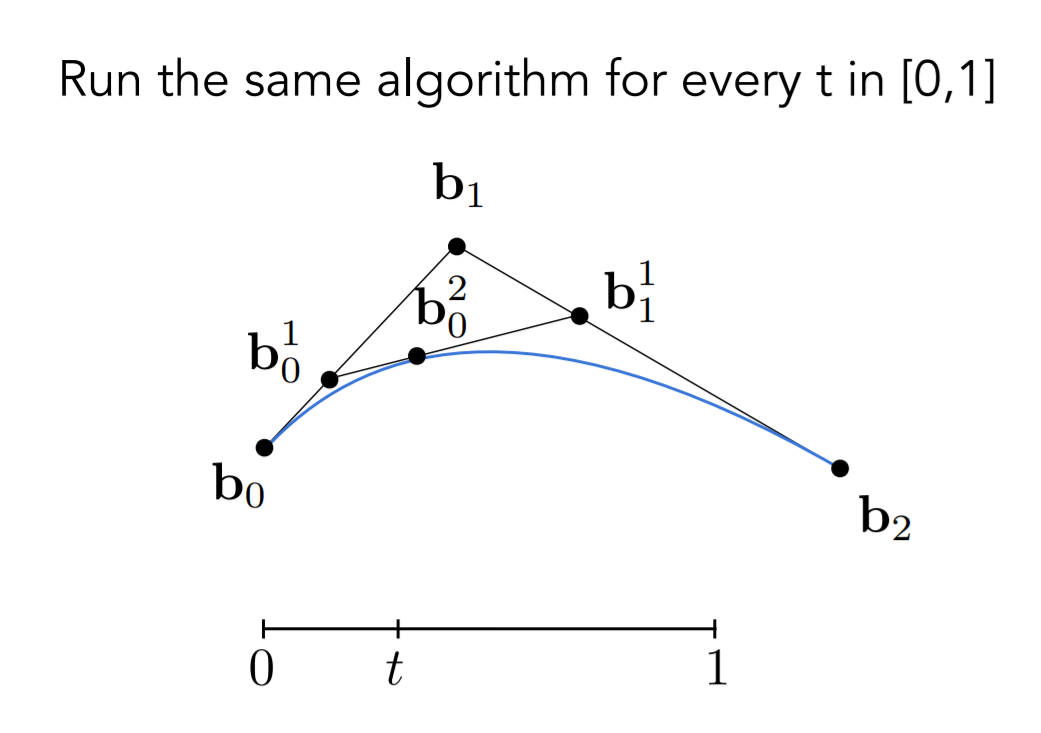（2）再考虑四个控制点，生成三次贝塞尔曲线。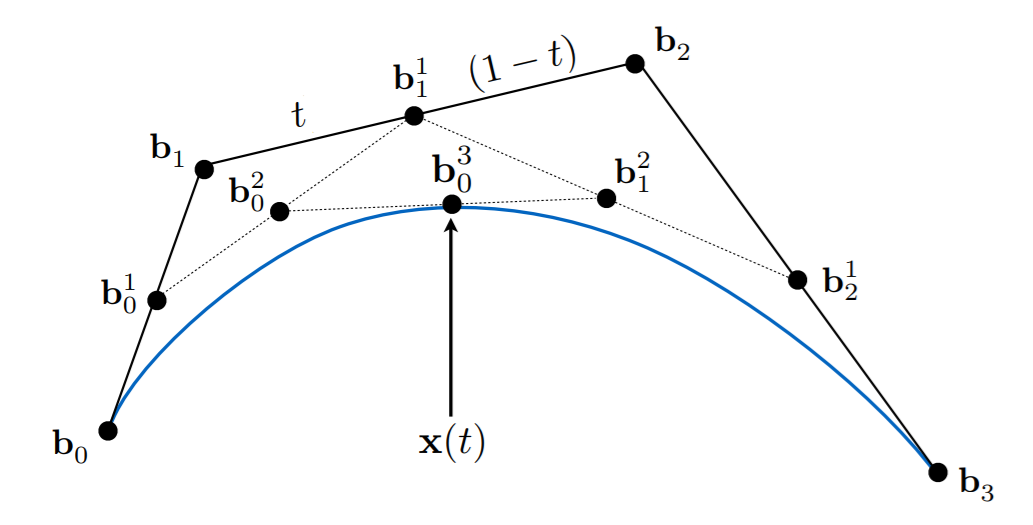(3) 代数形式：插值！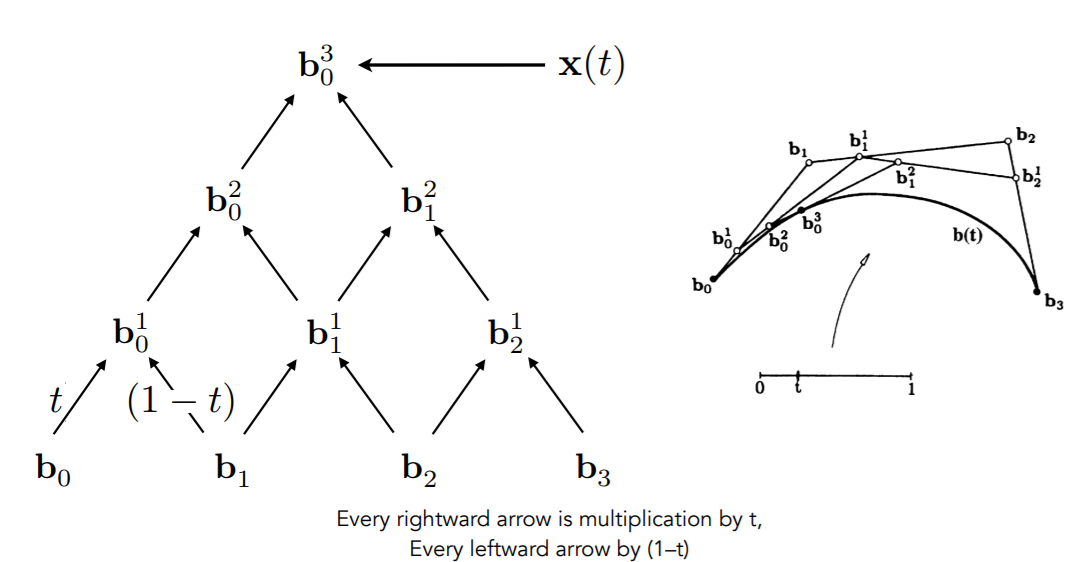### 实验结果## PA5

• Renderer.cpp 中的 Render()：这里你需要为每个像素生成一条对应的光线，然后调用函数 castRay() 来得到颜色，最后将颜色存储在帧缓冲区的相应像素中。
• Triangle.hpp 中的 rayTriangleIntersect(): v0, v1, v2 是三角形的三个顶点，orig 是光线的起点，dir 是光线单位化的方向向量。tnear, u, v 是你需要使用我们课上推导的 Moller-Trumbore 算法来更新的参数。

### 算法回顾

• Whitted-style Ray Tracing• Möller Trumbore 算法

Möller Trumbore 算法，可以直接判断交点是否在三角形内（需要用到克莱姆法则）：

### 实验结果## PA6

### 算法回顾

#### 包围盒求交#### BVH• 沿着最长的维度来划分
• 划分端点取中位数
• 取三角形的重心#### SAH 加速

（BVH）构建过程中最重要的问题就是如何对图元进行划分…划分时要尽可能减少划分后两部分包围盒重叠的体积，因为重叠的体积越大，光线穿过重叠区域的可能性越大，遍历两个子树的可能性就越高，计算消耗越多

• 取坐标轴跨度的中点 $t_{\operatorname{mid}}=\frac{t_{\max }+t_{\min }}{2}$​ ，若节点的坐标小于 $t_{mid}$ 则将其划分到左节点，否则将其划分到右节点（中点划分）。
• 最左边的 $\frac n 2$​ 个被划分到左节点，剩下的被划分到右节点（等量划分）。 // 作业框架中 Naive 的划分由此实现

SAH 考虑到了图元在空间中的分布也考虑到了子节点包围体的重叠程度，在实际应用中拥有很好的效果。

• 将物体按照质心顺序排序. $O(n \log n)$
• $\forall k$​，记录 $a_i := \cup_{i \le k} \ S(i)$​. $O(n)$​​
• $\forall k$​，记录 $b_i := \cup_{i \gt k} \ S(i)$​. $O(n)$​
• $\forall k$​，考虑划分 $[0, k] \ || \ [k+1, n)$​，计算其代价并更新最小代价. $O(n)$​

### 实验结果

// Warning: this code may be buggy!

// 没错用了这 ** 优化又慢了我也不知道x

// 我感觉包围盒算法就需要大改，anyway 领会精神吧x### 实验框架

• main.cpp
• 创建场景（1280x960）与预处理
• 导入模型为 MeshTriangle 类对象，并添加入场景；
• 将光源添加入场景；
• 对场景执行 buildBVH()
• 创建 Renderer 类对象渲染器，并开始渲染
• Vector.hpp, Vector.cpp：向量类，提供了向量 Vector3f, Vector2f 的基本操作
• Object.hpp：抽象类
• 提供了以下接口：
• bool intersect(const Ray&)
• bool intersect(const Ray& ray, float& distance, int& index) const
• Intersection getIntersection(Ray _ray)
• void getSurfaceProperties(const Vector3f &, const Vector3f &, const uint32_t &, const Vector2f &, Vector3f &, Vector2f &) const
• virtual Vector3f evalDiffuseColor(const Vector2f &) const
• virtual Bounds3 getBounds()
• 总结来说，首先是判断有无交点和获取交点的接口，然后是获取表面属性和计算颜色的接口，然后是获取包围盒的接口。
• Triangle.hpp/Sphere.hpp：继承自 Object，重载上述函数并实现为对应对象
• Ray.hpp
• Vector3f orig, direction;, double t, t_min, t_max;
• Light.hppVector3f position, intensity;
• AreaLight.hppVector3f u, v, normal; double length;
• Intersection.hpp
• bool happened;
• Vector3f coords, normal;
• double distance;
• Object* obj;
• Material* m;
• Material.hpp：存储表面属性
• 表面类型： enum MaterialType { DIFFUSE_AND_GLOSSY, REFLECTION_AND_REFRACTION, REFLECTION };
• 表面颜色（反射，辐射），折射率，BP 模型中的 $k_d, k_s, p$​
• Bounds3.hpp：包围盒相关函数
• 求对角线，维数，表面积，中心点；
• 与另外一个包围盒求交，求并；
• 检测两个包围盒是否互相包含或者重叠；
• 判断与光线是否相交；
• BVH.hpp/BVH.cpp：加速结构
• enum class SplitMethod { NAIVE, SAH };
• 与光线求交
• Intersection Intersect(const Ray &ray) const;
• Intersection getIntersection(BVHBuildNode* node, const Ray& ray)const;
• bool IntersectP(const Ray &ray) const;
• 本质是一颗二叉树，拥有树形结构
• BVHBuildNode* root;
• BVHBuildNode* recursiveBuild(std::vector<Object*>objects);
• BVHBuildNode* recursiveBuildSAH(std::vector<Object*>objects);
• 存储其图元 primitive 的指针
• std::vector<Object*> primitives;
• Renderer.hpp / Renderer.cpp
• 给定一个场景，创建渲染任务
• 管理任务过程中的 framebuffer 等等图像数据
• 管理整个渲染流程以及数据最终的写入文件
• Scene.hpp / Scene.cpp
• 图像相关的设定
• int width, height;
• double fov;
• Vector3f backgroundColor;
• maxDepth;
• 模型相关的设定 Get / Set
• void Add(Object *object);
• void Add(std::unique_ptr<Light> light);
• 与光线求交的函数：Intersection intersect(const Ray& ray) const;
• BVH 相关：
• BVHAccel *bvh = nullptr;
• void buildBVH();
• 渲染主函数：
• Vector3f castRay(const Ray &ray, int depth) const; // Important!
• bool trace(const Ray &ray, const std::vector<Object*> &objects, float &tNear, uint32_t &index, Object **hitObject);

## PA7

• 实现 Path Tracing 算法

### 算法回顾

def ray_generation(camPos, pixel):
Uniformly choose N samples from the pixel
for sample in the pixel:
Shoot a ray r(camPos, cam_to_sample)
if ray r hit the scene at p:

# Contributions from the light source
L_dir = 0.0
Uniformly sample the light at x' (pdf_light = 1/A)
Shoot a ray from p to x'
if the ray is not blocked in the middle:
L_dir = L_i * f_r * cos \theta * cos \theta' / |x'-p|^2 / pdf_light

# Contributions from other places
L_indir = 0.0
If test Russian Roulette with probability P_RR :
Uniformly sample the hemisphere toward wi (pdf_hemi = 1 / 2pi)
Trace a ray r(p, wi)
If ray r hit a non-emitting object at q:
L_indir = shade(q, -wi) * f_r * cos \theta / pdf_hemi / P_RR

Return L_dir + L_indir

### 实验结果

• SPP = 16 / 32 / 64 / 256## PA8

• 连接绳子约束，正确的构造绳子
• 半隐式欧拉法
• 显式欧拉法
• 显式 Verlet
• 阻尼

• 显式/半隐式欧拉法

• 显式 Verlet
• 阻尼